# quadpy Release 0.16.16

Numerical integration, quadrature for various domains

Keywords
mathematics, physics, engineering, quadrature, cubature, integration, numerical
GPL-3.0+/OML
Install
``` pip install quadpy==0.16.16 ```

### Documentation

Your one-stop shop for numerical integration in Python.

More than 1500 numerical integration schemes for line segments, circles, disks, triangles, quadrilaterals, spheres, balls, tetrahedra, hexahedra, wedges, pyramids, n-spheres, n-balls, n-cubes, n-simplices, the 1D half-space with weight functions exp(-r), the 2D space with weight functions exp(-r), the 3D space with weight functions exp(-r), the nD space with weight functions exp(-r), the 1D space with weight functions exp(-r2), the 2D space with weight functions exp(-r2), the 3D space with weight functions exp(-r2), and the nD space with weight functions exp(-r2), for fast integration of real-, complex-, and vector-valued functions.

For example, to numerically integrate any function over any given interval, install quadpy from the Python Package Index with

``````pip install quadpy
``````

and do

```import numpy as np

def f(x):
return np.sin(x) - x

This is like scipy with the addition that quadpy handles complex-, vector-, matrix-valued integrands, and "intervals" in spaces of arbitrary dimension.

To integrate over a triangle, do

```import numpy as np

def f(x):
return np.sin(x) * np.sin(x)

triangle = np.array([[0.0, 0.0], [1.0, 0.0], [0.7, 0.5]])

# get a "good" scheme of degree 10
val = scheme.integrate(f, triangle)```

Most domains have `get_good_scheme(degree)`. If you would like to use a particular scheme, you can pick one from the dictionary `quadpy.t2.schemes`.

All schemes have

```scheme.points
scheme.weights
scheme.degree
scheme.source
scheme.test_tolerance

scheme.show()
scheme.integrate(
# ...
)```

and many have

```scheme.points_symbolic
scheme.weights_symbolic```

quadpy is fully vectorized, so if you like to compute the integral of a function on many domains at once, you can provide them all in one `integrate()` call, e.g.,

```# shape (3, 5, 2), i.e., (corners, num_triangles, xy_coords)
triangles = np.stack(
[
[[0.0, 0.0], [1.0, 0.0], [0.0, 1.0]],
[[1.2, 0.6], [1.3, 0.7], [1.4, 0.8]],
[[26.0, 31.0], [24.0, 27.0], [33.0, 28]],
[[0.1, 0.3], [0.4, 0.4], [0.7, 0.1]],
[[8.6, 6.0], [9.4, 5.6], [7.5, 7.4]],
],
axis=-2,
)```

The same goes for functions with vectorized output, e.g.,

```def f(x):
return [np.sin(x), np.sin(x)]```

More examples under test/examples_test.py.

Read more about the dimensionality of the input/output arrays in the wiki.

## Schemes

### Line segment (C1)See here for how to generate Gauss formulas for your own weight functions.

Example:

```import numpy as np

scheme.show()
val = scheme.integrate(lambda x: np.exp(x), [0.0, 1.0])```

### 1D half-space with weight function exp(-r) (E1r)Example:

```import quadpy

scheme = quadpy.e1r.gauss_laguerre(5, alpha=0)
scheme.show()
val = scheme.integrate(lambda x: x**2)```

### 1D space with weight function exp(-r2) (E1r2)Example:

```import quadpy

scheme.show()
val = scheme.integrate(lambda x: x**2)```

### Circle (U2)• Krylov (1959, arbitrary degree)

Example:

```import numpy as np

scheme.show()
val = scheme.integrate(lambda x: np.exp(x), [0.0, 0.0], 1.0)```

### Triangle (T2)Apart from the classical centroid, vertex, and seven-point schemes we have

Example:

```import numpy as np

scheme.show()
val = scheme.integrate(lambda x: np.exp(x), [[0.0, 0.0], [1.0, 0.0], [0.5, 0.7]])```

### Disk (S2)Example:

```import numpy as np

scheme.show()
val = scheme.integrate(lambda x: np.exp(x), [0.0, 0.0], 1.0)```Example:

```import numpy as np

val = scheme.integrate(
lambda x: np.exp(x),
[[[0.0, 0.0], [1.0, 0.0]], [[0.0, 1.0], [1.0, 1.0]]],
)```

The points are specified in an array of shape (2, 2, ...) such that `arr` is the lower left corner, `arr` the upper right. If your c2 has its sides aligned with the coordinate axes, you can use the convenience function

`quadpy.c2.rectangle_points([x0, x1], [y0, y1])`

to generate the array.

### 2D space with weight function exp(-r) (E2r)Example:

```import quadpy

scheme.show()
val = scheme.integrate(lambda x: x ** 2)```

### 2D space with weight function exp(-r2) (E2r2)Example:

```import quadpy

scheme.show()
val = scheme.integrate(lambda x: x ** 2)```

### Sphere (U3)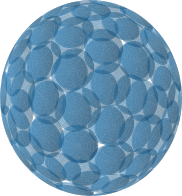Example:

```import numpy as np

# scheme.show()
val = scheme.integrate(lambda x: np.exp(x), [0.0, 0.0, 0.0], 1.0)```

Integration on the sphere can also be done for functions defined in spherical coordinates:

```import numpy as np

def f(theta_phi):
theta, phi = theta_phi
return np.sin(phi) ** 2 * np.sin(theta)

val = scheme.integrate_spherical(f)```

### Ball (S3)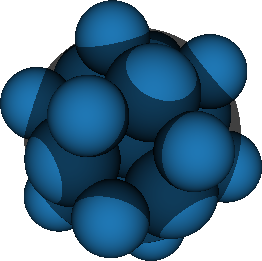Example:

```import numpy as np

# scheme.show()
val = scheme.integrate(lambda x: np.exp(x), [0.0, 0.0, 0.0], 1.0)```

### Tetrahedron (T3)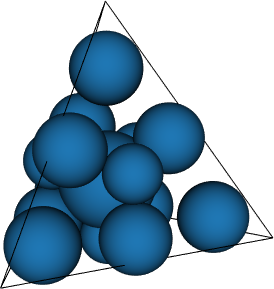Example:

```import numpy as np

# scheme.show()
val = scheme.integrate(
lambda x: np.exp(x),
[[0.0, 0.0, 0.0], [1.0, 0.0, 0.0], [0.5, 0.7, 0.0], [0.3, 0.9, 1.0]],
)```

### Hexahedron (C3)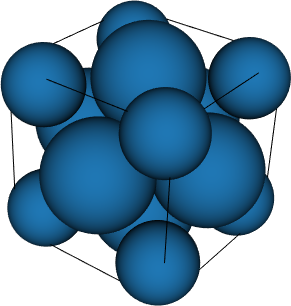Example:

```import numpy as np

# scheme.show()
val = scheme.integrate(
lambda x: np.exp(x),
quadpy.c3.cube_points([0.0, 1.0], [-0.3, 0.4], [1.0, 2.1]),
)```

### Pyramid (P3)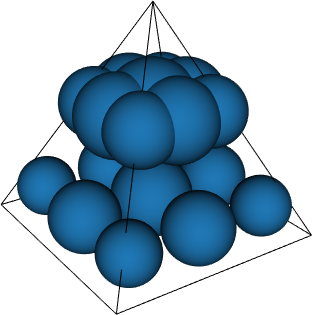• Felippa (2004, 9 schemes up to degree 5)

Example:

```import numpy as np

val = scheme.integrate(
lambda x: np.exp(x),
[
[0.0, 0.0, 0.0],
[1.0, 0.0, 0.0],
[0.5, 0.7, 0.0],
[0.3, 0.9, 0.0],
[0.0, 0.1, 1.0],
],
)```

### Wedge (W3)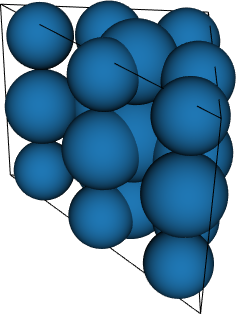Example:

```import numpy as np

val = scheme.integrate(
lambda x: np.exp(x),
[
[[0.0, 0.0, 0.0], [1.0, 0.0, 0.0], [0.5, 0.7, 0.0]],
[[0.0, 0.0, 1.0], [1.0, 0.0, 1.0], [0.5, 0.7, 1.0]],
],
)```

### 3D space with weight function exp(-r) (E3r)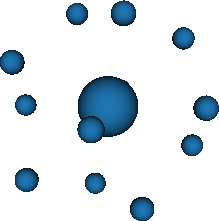Example:

```import quadpy

# scheme.show()
val = scheme.integrate(lambda x: x ** 2)```

### 3D space with weight function exp(-r2) (E3r2)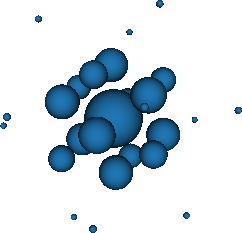Example:

```import quadpy

# scheme.show()
val = scheme.integrate(lambda x: x ** 2)```

### n-Simplex (Tn)

Example:

```import numpy as np

dim = 4
scheme = quadpy.tn.grundmann_moeller(dim, 3)
val = scheme.integrate(
lambda x: np.exp(x),
np.array(
[
[0.0, 0.0, 0.0, 0.0],
[1.0, 2.0, 0.0, 0.0],
[0.0, 1.0, 0.0, 0.0],
[0.0, 3.0, 1.0, 0.0],
[0.0, 0.0, 4.0, 1.0],
]
),
)```

### n-Sphere (Un)

Example:

```import numpy as np

dim = 4
val = scheme.integrate(lambda x: np.exp(x), np.zeros(dim), 1.0)```

### n-Ball (Sn)

Example:

```import numpy as np

dim = 4
val = scheme.integrate(lambda x: np.exp(x), np.zeros(dim), 1.0)```

### n-Cube (Cn)

Example:

```import numpy as np

dim = 4
val = scheme.integrate(
lambda x: np.exp(x),
quadpy.cn.ncube_points([0.0, 1.0], [0.1, 0.9], [-1.0, 1.0], [-1.0, -0.5]),
)```

### nD space with weight function exp(-r) (Enr)

Example:

```import quadpy

dim = 4
```import quadpy